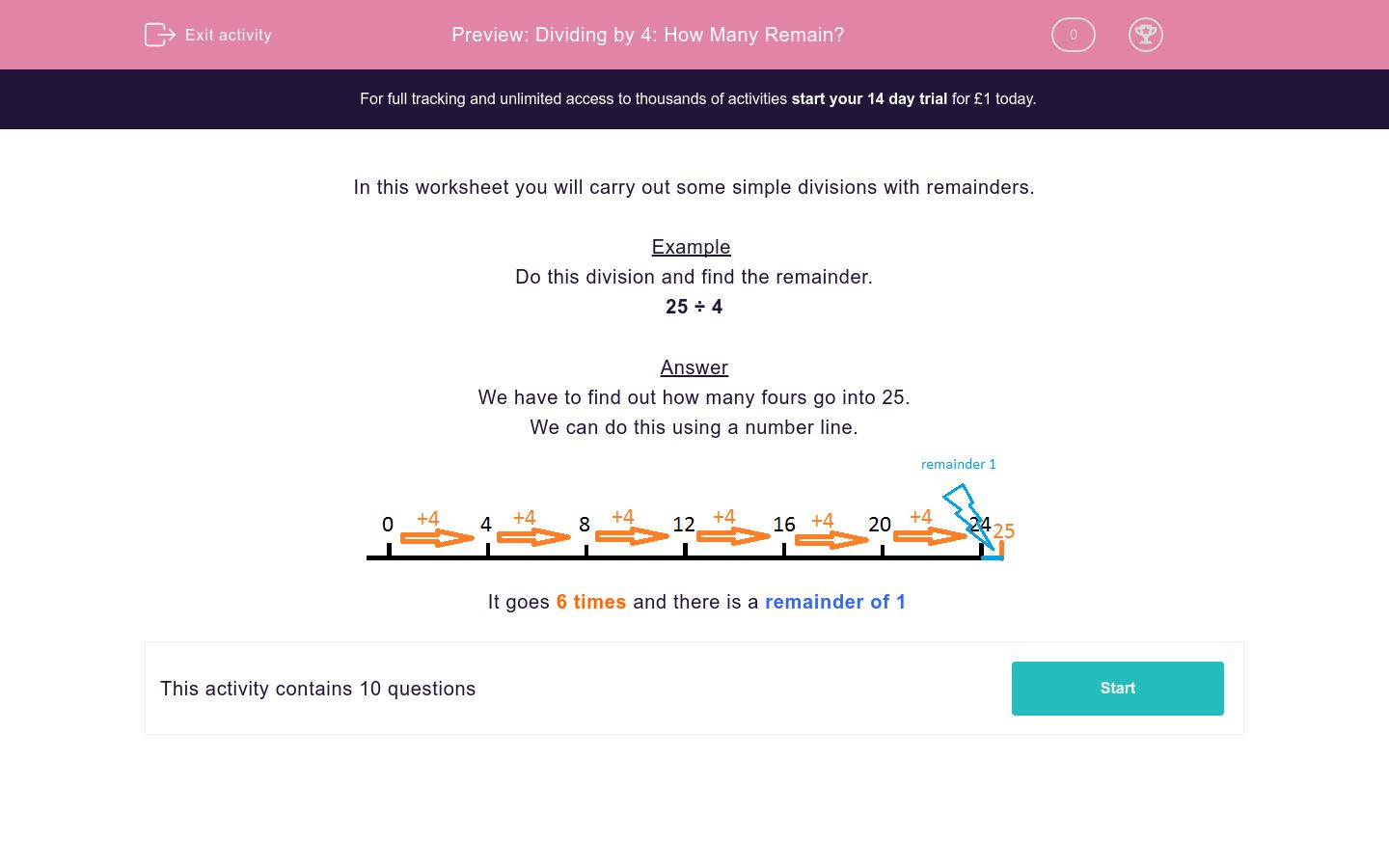# Dividing by 4: How Many Remain?

In this worksheet, students divide two-digit numbers by 4 and note the remainders.Key stage:  KS 2

Curriculum topic:   Maths and Numerical Reasoning

Curriculum subtopic:   Mixed Problems

Difficulty level:### QUESTION 1 of 10

In this worksheet you will carry out some simple divisions with remainders.

Example

Do this division and find the remainder.

25 ÷ 4

We have to find out how many fours go into 25.

We can do this using a number line.It goes 6 times and there is a remainder of 1

Work out the division below.

17  ÷  4  =
 A
remainder
 B

## Column B

A
4
B
1

Work out the division below.

35  ÷  4  =
 A
remainder
 B

## Column B

B
3
A
8

Work out the division below.

37  ÷  4  =
 A
remainder
 B

## Column B

B
9
A
1

Work out the division below.

50  ÷  4  =
 A
remainder
 B

## Column B

A
12
B
2

Work out the division below.

32  ÷  4  =
 A
remainder
 B

## Column B

A
8
B
0

Work out the division below.

59  ÷  4  =
 A
remainder
 B

## Column B

A
14
B
3

Work out the division below.

42  ÷  4  =
 A
remainder
 B

## Column B

B
2
A
10

Work out the division below.

23  ÷  4  =
 A
remainder
 B

## Column B

B
3
A
5

Work out the division below.

19  ÷  4  =
 A
remainder
 B

## Column B

B
3
A
4

Work out the division below.

82  ÷  4  =
 A
remainder
 B

## Column B

B
2
A
20
• Question 1

Work out the division below.

17  ÷  4  =
 A
remainder
 B

## Column B

A
4
B
1
• Question 2

Work out the division below.

35  ÷  4  =
 A
remainder
 B

## Column B

B
3
A
8
• Question 3

Work out the division below.

37  ÷  4  =
 A
remainder
 B

## Column B

B
1
A
9
• Question 4

Work out the division below.

50  ÷  4  =
 A
remainder
 B

## Column B

A
12
B
2
• Question 5

Work out the division below.

32  ÷  4  =
 A
remainder
 B

## Column B

A
8
B
0
• Question 6

Work out the division below.

59  ÷  4  =
 A
remainder
 B

## Column B

A
14
B
3
• Question 7

Work out the division below.

42  ÷  4  =
 A
remainder
 B

## Column B

B
2
A
10
• Question 8

Work out the division below.

23  ÷  4  =
 A
remainder
 B

## Column B

B
3
A
5
• Question 9

Work out the division below.

19  ÷  4  =
 A
remainder
 B

## Column B

B
3
A
4
• Question 10

Work out the division below.

82  ÷  4  =
 A
remainder
 B

## Column B

B
2
A
20
---- OR ----